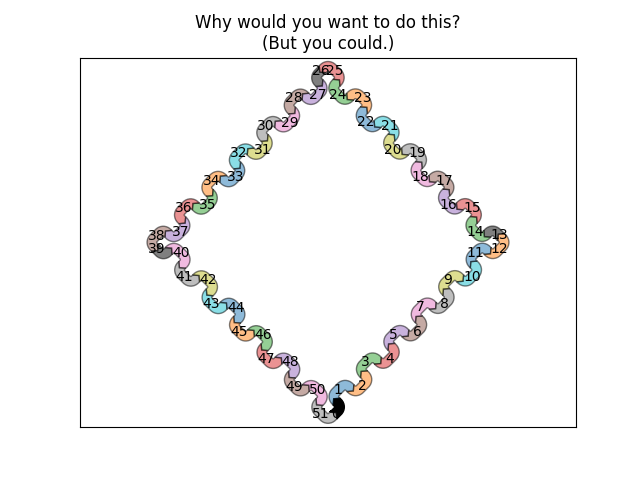# Long chain of connections using Sankey¶

Demonstrate/test the Sankey class by producing a long chain of connections.

import matplotlib.pyplot as plt
from matplotlib.sankey import Sankey

links_per_side = 6

def side(sankey, n=1):
"""Generate a side chain."""
prior = len(sankey.diagrams)
for i in range(0, 2*n, 2):
sankey.add(flows=[1, -1], orientations=[-1, -1],
patchlabel=str(prior + i),
prior=prior + i - 1, connect=(1, 0), alpha=0.5)
sankey.add(flows=[1, -1], orientations=[1, 1],
patchlabel=str(prior + i + 1),
prior=prior + i, connect=(1, 0), alpha=0.5)

def corner(sankey):
"""Generate a corner link."""
prior = len(sankey.diagrams)
sankey.add(flows=[1, -1], orientations=[0, 1],
patchlabel=str(prior), facecolor='k',
prior=prior - 1, connect=(1, 0), alpha=0.5)

fig = plt.figure()
ax = fig.add_subplot(1, 1, 1, xticks=[], yticks=[],
title="Why would you want to do this?\n(But you could.)")
sankey = Sankey(ax=ax, unit=None)
sankey.add(flows=[1, -1], orientations=[0, 1],
patchlabel="0", facecolor='k',
rotation=45)
side(sankey, n=links_per_side)
corner(sankey)
side(sankey, n=links_per_side)
corner(sankey)
side(sankey, n=links_per_side)
corner(sankey)
side(sankey, n=links_per_side)
sankey.finish()
# Notice:
# 1. The alignment doesn't drift significantly (if at all; with 16007
#    subdiagrams there is still closure).
# 2. The first diagram is rotated 45 deg, so all other diagrams are rotated
#    accordingly.

plt.show()